### Home > PC > Chapter 8 > Lesson 8.2.3 > Problem8-111

8-111.
1. THE RULE OF 70 Homework Help ✎

The Rule of 70 is a useful rule of thumb for determining the doubling time under steady (exponential) growth. To get the doubling time, the rule tells us to divide 70 by the growth rate, expressed as a percentage. Carry out the following steps to see why the Rule of 70 works as it does.

1. Use A = Pert with A = 2P to find the exact doubling time t in terms of the growth rate r without using your calculator. In other words express t in terms of r.

2. If r is the rate of growth (i.e. 0.07), and R is the percentage rate of growth (i.e. 7%), express r in terms of R.

3. Using your calculator to evaluate ln 2, show that doubling time with growth rate is t ≈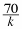.

4. 100 ln 2 is closer to 69 than 70. Why do we use 70?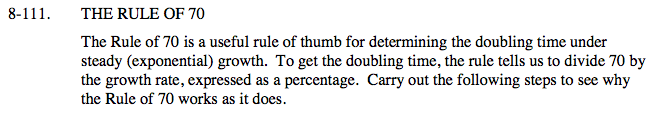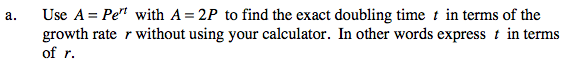2P = Pert

$t=\frac{\text{ln}2}{r}$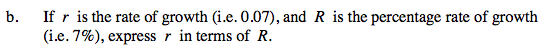$r=\frac{R}{100}$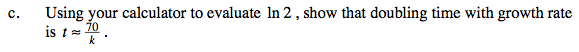$t=\frac{\text{ln}2}{r} \ \ \ t=\frac{.6931}{\frac{R}{100}} \ \ \text{ Then simplify.}$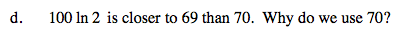We use 70 because it makes the mental arithmetic easier.